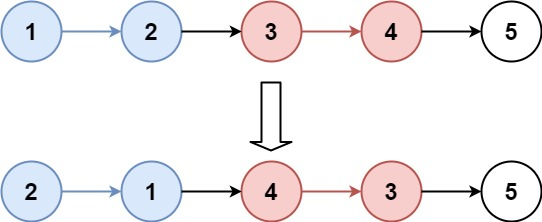# Reverse Nodes in k-Group LeetCode Solution

Frequently asked in Adobe Amazon Apple Bloomberg ByteDance Capital One eBay Intuit MakeMyTrip Microsoft Oracle Snapchat Tencent Uber VMware Yahoo Zenefits
Walmart ZoomViews 97

## Problem Statement:

Reverse Nodes in k-Group LeetCode Solution – Given the `head` of a linked list, reverse the nodes of the list `k` at a time, and return the modified list.

`k` is a positive integer and is less than or equal to the length of the linked list. If the number of nodes is not a multiple of `k` then left-out nodes, in the end, should remain as it is.

You may not alter the values in the list’s nodes, only nodes themselves may be changed.

Example 1:Input:

``` head = [1,2,3,4,5], k = 2
```

Output:

``` [2,1,4,3,5]
```

Example 2:Input:

``` head = [1,2,3,4,5], k = 3
```

Output:

``` [3,2,1,4,5]
```

Constraints:

• The number of nodes in the list is `n`.
• `1 <= k <= n <= 5000`
• `0 <= Node.val <= 1000`

Follow-up: Can you solve the problem in `O(1)` extra memory space?

## ALGORITHM –

### IDEA –

• In order to Reverse Nodes in k-Group LeetCode Solution. First, we will focus on how to reverse node in the k group so at first, we will count the total length of the linked list.
• Then we will reverse the linked list according to the given size ‘k’ and reverse the linked list.
• After that, we will decrease the total length by k and will check if the length of the linked list is greater than or equal to the size then we will repeat the previous steps by using recursion.
• Else we will link the linked list with the remaining node.
• Hence we will reverse the linked list in the k-group.

### APPROACH –

• First, we will make a variable total(length of linked list). after that will count the total length by using the while loop.
• Then we will reverse the given linked list according to size – next – (next of current element), prev – ( previous element of current), and current.
• Then we will decrease the total length by k after reversing the linked list and will check if the total length will be greater or equal to k then we will do the previous steps by using recursion.
• Else will link to the remaining node of the linked list.

## Image –```class Solution:
def reverseKGroup(self, head: Optional[ListNode], k: int) -> Optional[ListNode]:

total = 0

while(current):
total += 1
current = current.next

prev = None
count = 0
while(current and count < k ):
next = current.next
current.next = prev
prev = current

current = next
count += 1

total -= k
if total >= k:

else:

return prev```
```class Solution {
public ListNode reverseKGroup(ListNode head, int k) {

int total = 0;
while(current != null){

total++;
current = current.next;
}
int count = 0;
ListNode prev = null;
ListNode next = null;
while(c != null && count < k){

next = c.next;
c.next = prev;
prev = c;
c = next;
count++;

}

total -= k;
if(total >= k){
}
else{
}

return prev;

}
}```

## Complexity Analysis for Reverse Nodes in k-Group LeetCode Solution

Time Complexity: O(N), As we have traversed the LinkedList three times.

Space Complexity: O(1), As we haven’t taken any extra space.

Translate »